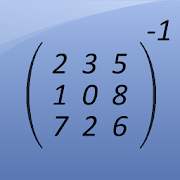# Matrix Inversion Calculator - Lumos Educational App Store3.91
Price - \$fREE

#### DESCRIPTION:

This free app is a math calculator, which is able to calculate the invertible of a matrix. The following matrices can be inverted: - 2x2 matrices - 3x3 matrices - 4x4 matrices Best math tool for school and college! If you are a student, it will helps you to learn linear algebra! Note: The inverse of a matrix A is the matrix B, with AxB=I where I is the identity matrix and the multiplication used is ordinary matrix multiplication. A matrix is a nonsingular matrix if it is an invertible matrix, and the determinant of this matrix does not equal 0.

#### OVERVIEW:

Matrix Inversion Calculator is a free educational mobile app By .It helps students in grades HS practice the following standards HSN.VM.C.10.

This page not only allows students and teachers download Matrix Inversion Calculator but also find engaging Sample Questions, Videos, Pins, Worksheets, Books related to the following topics.

1. HSN.VM.C.10 : Understand that the zero and identity matrices play a role in matrix addition and multiplication similar to the role of 0 and 1 in the real numbers. The determinant of a square matrix is nonzero if and only if the matrix has a multiplicative inverse..

HS

#### STANDARDS:

HSN.VM.C.10

Developer:

Software Version: 3.7

Category:

### RELATED APPSEdSearch WebSearch